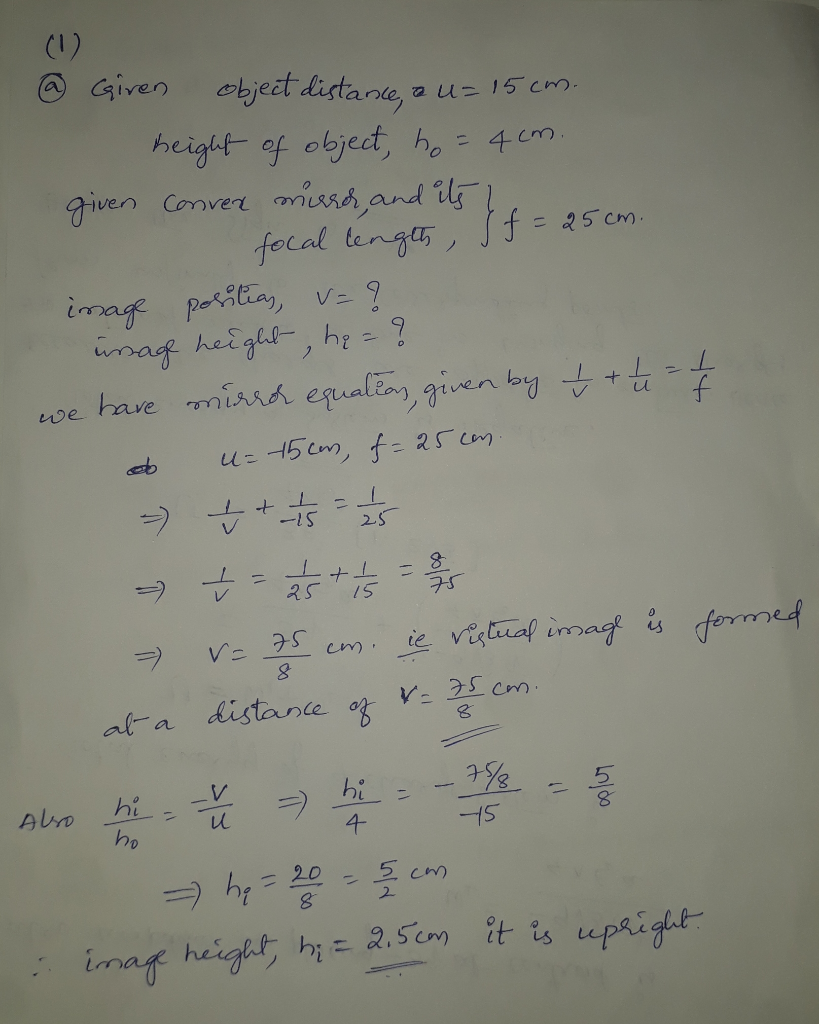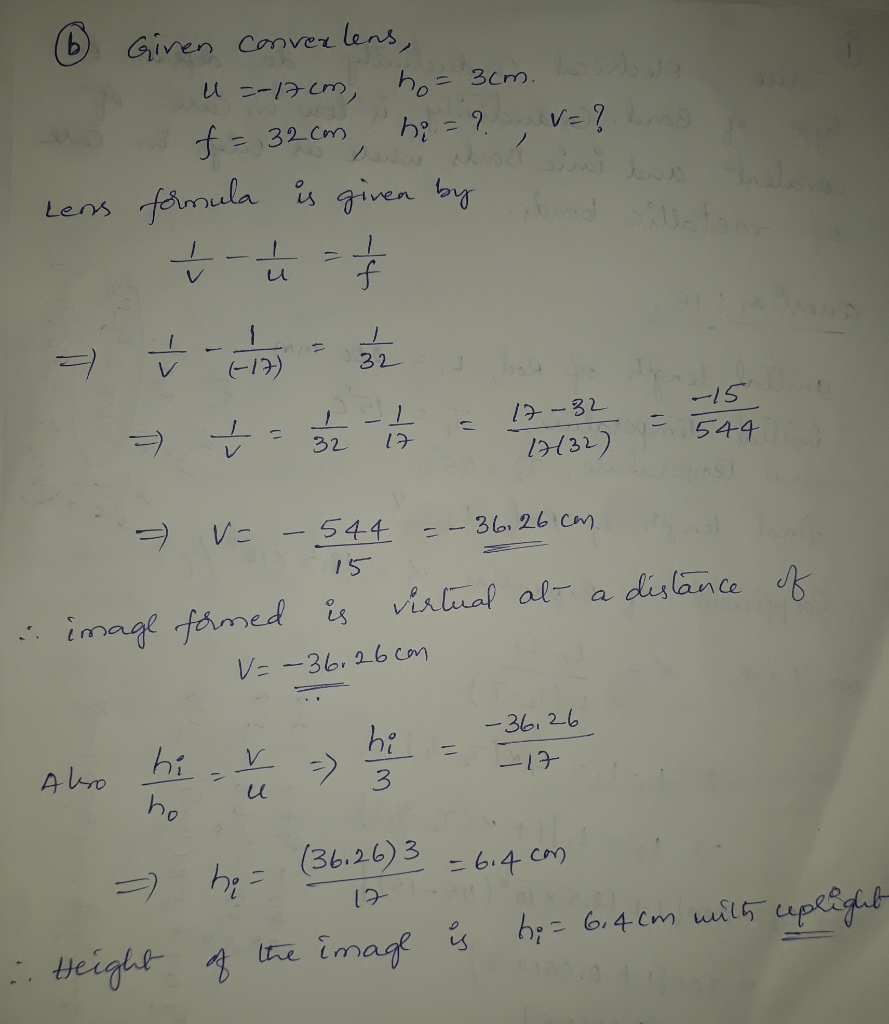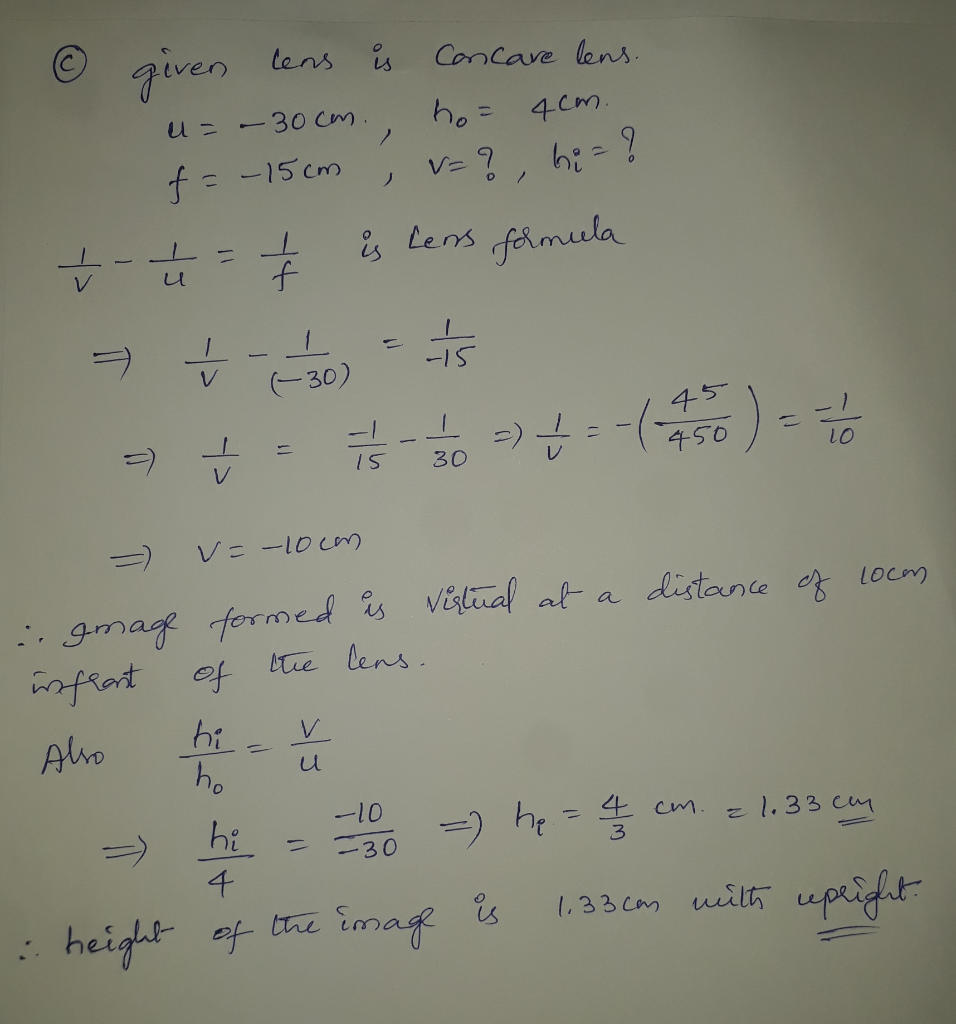In: Physics

# a) A 4.0 cm -tall object is 15 cm in front of a CONVEX mirror that has a -25 cm focal length.

1. Please complete the following parts of the question regarding optics.

a) A 4.0 cm -tall object is 15 cm in front of a CONVEX mirror that has a -25 cm focal length. Calculate the image position then Calculate the image height. Type a positive value if the image is upright and a negative value if it is inverted.

b) A 3.0-cm-tall object is 17 cm in front of a CONVERGING lens that has a 32 cm focal length. Calculate the image position then Calculate the image height. Type a positive value if the image is upright and a negative value if it is inverted.

c) A 4.0-cm-tall object is 30 cm in front of a diverging lens that has a -15 cm focal length. Calculate the image position then Calculate the image height. Type a positive value if the image is upright and a negative value if it is inverted.

## Solutions

##### Expert Solution## Related Solutions

##### A 4.7 cm tall object is placed 32 cm in front of a spherical mirror. It is desired to produce a virtual image that is upright and 3.3 cm tall.
CE CHP 22 QS 13A 4.7 cm tall object is placed 32 cm in front of a spherical mirror. It is desired to produce a virtual image that is upright and 3.3 cm tall.Part BWhere is the image located?Part CWhat is the focal length of the mirror?Part DWhat is the radius of curvature of the mirror?
##### An object is placed 31.5 cm from a +5.0-D lens. A spherical mirror with focal length...
An object is placed 31.5 cm from a +5.0-D lens. A spherical mirror with focal length 25 cm is placed 75 cm behind the lens. Note that the mirror reflects light back through the lens. Find the distance from original object to the final image, measured from the object toward the lens. Enter negative value if the object is between the image and the mirror and positive value otherwise.
##### Consider a double convex lens with a focal length of 35 cm. If a marble is...
Consider a double convex lens with a focal length of 35 cm. If a marble is placed 85 cm from the lens, what is the image distance? Enter your answer in cm.
##### An object 20 cm tall is placed at 50 cm in front of a converging lens...
An object 20 cm tall is placed at 50 cm in front of a converging lens with a focal length of 5 cm. A. Find the location and nature of the image. B. Draw the diagram
##### When an object is placed at a distance greater than the focal length but less than twice the focal length from a convex lense
When an object is placed at a distance greater than the focal length but less than twice the focal length from a convex lense the image is diminished.the image is virtual.All of the other choices are not correct.the image is inverted.the image is formed on the same side as theobject.For an object placed in front of a concave lense,the image is always realthe image may be erect or inverted.the image is formed on the other side of thelense.the image may be...
##### When an object is placed 10 cm in front of a mirror, an image is formed...
When an object is placed 10 cm in front of a mirror, an image is formed 45 cm behind the mirror. What is the mirror’s focal length?
##### ​A concave cosmetic mirror has a focal length of 24 cm . A 3.0-cm-long mascara brush is held upright 12 cm from the mirror.
A concave cosmetic mirror has a focal length of 24 cm . A 3.0-cm-long mascara brush is held upright 12 cm from the mirror.Part AUse ray tracing to determine the location of its image.Part BWhat is the height of the image? Use ray tracing to determine.
##### The tube length in a certain compound microscope is 25 cm. The focal length of the...
The tube length in a certain compound microscope is 25 cm. The focal length of the objective lens is 2.1 cm. The clearest image is formed when an object of height 1 mm is placed at a distance of 2.3 cm from the objective lens. Determine: (i) The position of the intermediate image, (ii) the focal length of the eye piece, that will place the final image at the near-point, (iii) the total magnification of the microscope and the height...
##### Two converging lenses, each of focal length 15 cm, are placed 52 cm apart, and an...
Two converging lenses, each of focal length 15 cm, are placed 52 cm apart, and an object is placed 30 cm in front of the first lens (a) Where is the final image formed with respect to the second lense? 60.8 cm behind the second lense 60.8 cm in front of the second lense 47.1 cm in front of the second lense 47.1 cm behind the second lense (b) What is the magnification of the system? 0.5 –1 –3.1 2.1
##### A convex, or converging, lens with a focal length of 34 cm is used to observe an image of an apple than is 80 cm away from the lens.
Part A A convex, or converging, lens with a focal length of 34 cm is used to observe an image of an apple than is 80 cm away from the lens. At what distance from the lens does the image form and what type of image is it? 46 cm, virtual 46 cm, real 59 cm, real 59 cm, virtual 24 cm, real 24 cm, virtual  Part B A person's eye has a diameter of 2.5 cm and a converging lens that produces a clear, real image on the retina....